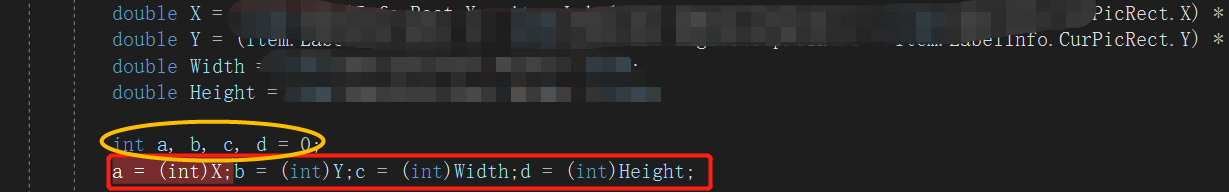• 仅作为记录，大佬请跳过。 用(int)X 先初始化变量，再用 int a, b, c, d = 0; a = (int)X;b = (int)Y;c = (int)Width;d = (int)Height;
仅作为记录，大佬请跳过。
用(int)X
先初始化变量，再用
int a, b, c, d = 0;
a = (int)X;b = (int)Y;c = (int)Width;d = (int)Height;展开全文• 在本文中，我们将看到如何将double转换int。 在Java编程中，您将有一个double原语值（例如82.14），但是要执行进一步的操作，您需要一个int值（例如82），所以让我们看看如何在Java中将double转换int。 您可以...
在本文中，我们将看到如何将double转换为int。
在Java编程中，您将有一个double原语值（例如82.14），但是要执行进一步的操作，您需要一个int值（例如82），所以让我们看看如何在Java中将double转换为int。
您可以通过三种方式将double转换为int。我将在下面列出所有内容，然后我们将一一列出。
1，将double转换为int —使用类型转换 2，将double转换为int —使用 Math.round() 3，将double转换为int —使用 Double.IntValue()
1.将double转换为int —使用类型转换
我们知道double是64位原始值，而int是32位原始值。因此，要将double转换为int，我们可以将double值下转换为int。
我在下面给出了一个简单的示例，该示例显示了使用类型转换将double转换为int的情况。
/**
* 一个使用typecasting将double转换为int的Java程序
**/
public class DoubleToIntUsingTypecasting{
public static void main(String []args){
double doubleValue = 82.14; // 82.14
System.out.println("doubleValue: "+doubleValue);
//typecase double to int
int intValue = (int) doubleValue; // 82
System.out.println("intValue: "+intValue);
}
}

输出：
doubleValue：82.14
整数值：82

类型转换的问题是它将截断小数点后的值。它不会四舍五入。
在82.14的情况下，我们将得到一个int值82，看起来不错。但是，当我们有一个像82.99这样的双精度值时，我们只会得到82，而失去了0.99，即〜1。
它可能会在您的计算中产生问题。
对于82.99，应将其四舍五入为83，然后转换为int。
类型转换是不可能的，但是我们的下一个解决方案可以实现它。
2.将double转换为int —使用 Math.round()
Math.round()方法会将浮点值四舍五入到最接近的long值。然后我们可以将其转换为int类型。
我在下面给出了一个简单的Java程序，该程序显示了如何使用Math.round()方法将double转换为int 。
/ **
*使用java将double转换为int的Java程序
* Math.round（）方法
** /
public class DoubleToIntUsingRoundMethod{
public static void main(String []args){
// 情况1
double doubleValue = 82.14; // 82.14
System.out.println("doubleValue: "+doubleValue);
//将case双精度型转换为int
int intValue = (int) Math.round(doubleValue); // 82
System.out.println("intValue: "+intValue);
System.out.println();
// 情况2
double nextDoubleValue = 82.99; //
System.out.println("nextDoubleValue: "+nextDoubleValue);
// Math.round（nextDoubleValue）返回long值
//将case的类型转换为int
int nextIntValue = (int) Math.round(nextDoubleValue);    // 83
System.out.println("nextIntValue: "+nextIntValue);
}
}

输出：
doubleValue：82.14
整数值：82
nextDoubleValue：82.99
nextIntValue：83

3.将double转换为int —使用 Double.IntValue()
通过这种方式，我们将double原语值转换为Doublewrapper类，然后可以使用wrapper类的intValue()方法Double。
此方法不会在将值转换为int值之前将其四舍五入。它将删除小数点后的数字。
我在下面给出了一个简单的Java程序，该程序显示了如何使用Double.IntValue()方法将double转换为int 。
/ **
*
*使用java将double转换为int的Java程序
* Double.intValue（）方法
*
** /
public class DoubleToIntUsingIntValueMethod{
public static void main(String []args){
double doubleValue = 82.14; // 82.14
System.out.println("doubleValue: "+doubleValue);
//创建Double wrapper对象
Double doubleValueObject = new Double(doubleValue);
//将case双精度型转换为int
int intValue = doubleValueObject.intValue(); // 82
System.out.println("intValue: "+intValue);
}
}

输出：
doubleValue：82.14
整数值：82

结论
我们可以使用下面给出的三种方式在java中将double转换为int。
1.使用doublecasting将double转换为int
在这种方法中，我们将double值强制转换为int，如下所示：
int  intValue  =（int）数学。舍入（doubleValue）;

但是这样，我们将失去小数点后的值。在将double转换为int之前，它将不进行舍入。
2.将double转换为int —使用 Math.round()
这样，我们将该Math.round()方法用于舍入目的。
Math.round() 方法将double值四舍五入到最接近的long，然后可以按如下所示将long类型转换为int。
int  nextIntValue  =（int）数学。回合（nextDoubleValue）;

3.将double转换为int —使用 Double.IntValue()
这样，我们将double值转换为Double包装器类，然后使用该Double.IntValue()方法获取int值。
//创建Double wrapper对象
Double  doubleValueObject  = 新 Double（doubleValue）;
//将case双精度型转换为int
int  intValue  =  doubleValueObject。intValue（）;

这样，我们还将丢失小数点后的数字。
因此，这就是我们在Java中将double转换为int的方式。
零基础学习Java编程技术，欢迎加入我的十年Java学习园地，
展开全文java
• double型可以强制转换成int型，即使不加强制转换，也会隐式转换，只是转换结果会丢弃小数部分，如：double d=1.6;int i;i=d ; //隐式转换，i结果为1i=(int)d ; //强制转换，i结果为1但，如果double变量数据，超过...

double型可以强制转换成int型，即使不加强制转换，也会隐式转换，只是转换结果会丢弃小数部分，如：double d=1.6;int i;i=d ; //隐式转换，i结果为1i=(int)d ; //强制转换，i结果为1但，如果double变量数据，超过整形数据的表示范围，（一般整数占四个字节，有符号数表示范围为：-2^31 ~ 2^31-1 )，则转换后的结果会是错误的。

转载于:https://www.cnblogs.com/zhangshuyao/p/8010760.html
展开全文• public static void main(String[] args) { double db = 15.87; int in = new Double(db).intValue(); System.out.println(in); } 运行结果： 15
• public class test09 { public static void main(String[] args) { double a = 5000.44; double b = 100.12; double v = a / b; int i = new Double(v).intValue(); ...
• doubleint类型会丢失精度,今天我们就回答为什么会丢失精度,以及最好的解决方案.C
• import java.math.BigDecimal; BigDecimal balance =(BigDecimal)getbalance_Map.get(“balance”); balance.intValue();转int int intBalance=balance==null?0:balance.intValue();...转double...java object
• Double d = Double.parseDouble(object.toString()); //转int int intBalance=d==null?0:d.intValue(); //转double Double d = Double.parseDouble(object.toString()); double intBalance=d==null?0:d;
• 从控制台接收到的用户输入的内容都是string类型，如果要进行计算，就需要将接收到的内容转换成数值类型 什么是隐式转换？如下图 什么是强制转换？如下图 一、代码展示 1.隐式转换：int——>double ...c#
• c++ string与常用数值变量互转 ...以下函数自动判断int或者double。 测试环境： 系统：win7或者win10 IDE：vs2015 本文包含的内容： c++ string与常用数值变量互转 1. 头文件 2. int转...C++ string
• Given a double type of variable, we have to convert it into an integer in C#. 给定双重类型的变量，我们必须在C＃中将其转换为... int_variable = (int)double_variable; Example: 例： Input: double...
• doubleint的快速转换  (2014-01-19 13:39:56) 转载▼ 标签：  double   long   数值类型转换   快速   图形表示用double，显示用int，很常规的提高效率的小...
• 标题Java类型转换: intdouble 由于double的范围比int数据类型大，所以当int值被赋给double时，java会自动将int转换double。 隐式转换：由于double数据类型的范围和内存大小都比int大，因此从intdouble的...
•string c语言 sstream
• while ((put = str.ReadLine()) != null) { strArray = put.Trim().ToString().Split(" ".ToCharArray()); rowsArray = Array.ConvertAll<string ,double>(strArray,delegate(string s){return doubl...c# array
• 今天小编就为大家分享一篇Android String类型转换为float、doubleint的工具类方法，具有很好的参考价值，希望对大家有所帮助。一起跟随小编过来看看吧
• 使用情景：后端返回的json字符串，通过gson处理后，原本是int类型，现在获取到的是double类型的。 解决办法： Map&lt;String,Object&gt; map = new Map&lt;String,Object&gt;; Gson gson = new ...
• 大家可能遇到这样的情况：double x=2.555;int y=(int)(x*1000);cout 结果:y=2554。如果要准确得到整型数据，可以这样。int y=(int)(x*1000.0); 或者是通过字符串转换。char ch;sprintf(ch,"%01f",x*1000);y=...
• 例子1（double型强制转换成int型）： 直接舍掉小数，只留下整数。 正数： double d = 5.5; int i = (int)d; System.out.println("i=："i); //输出5 double b = 5.9; int i1 = (int)b; System.out....取整
• 今天在计算数据的时候，我用c++算数的时候出现了点问题，如下图，就是double 类型的d取值为1，然后N为整型20，densityC为宏定义的0.5， 结果算出来1.0\*20\*0.5得出数值为9？？？这计算机不拿我当人呢，请问大佬们...c++ c语言 开发语言
• C＃Convert.ToInt32(double)方法 (C# Convert.ToInt32(double) Method) Convert.ToInt32(double) Method is used to convert a specific double value (a double precision floating point number) to its ...c# java python c++ .net
• // 字节到浮点转换public static double byteToDouble(byte[] b,int offset) {long l;l = b[offset+0] & 0xff;l |= (((long) (b[offset+1] & 0xff)) );l |= (((long) (b[offset+2] & 0xff)) );l |= (((long) (b[of
• 本文主要介绍Java中double数据类型变量，转换成int数组的方法、字符串转成 int数组的方法、int数组转成字符串的方法。 原文地址：Java double数据类型转换成int数组的方法
• ## double转int问题

千次阅读 2018-09-03 16:15:34
一、代码实现 ... public class TTTT { public static void main(String[] args) { double d =1.76; System.out.println((int)d); double f =1.16; System.out.println((int)f); } ...java se
• double t = 3.9; int x = (int)(t + 0.5);// 当 t &gt; 0.5时 可以避免转换成3 cout &lt;&lt; x; // 输出为: 4
• public class Double2Int { /** * @param args */ public static void main(String[] args) { // TODO Auto-generated method stub double d = 5.5; int i = (int)d; ......